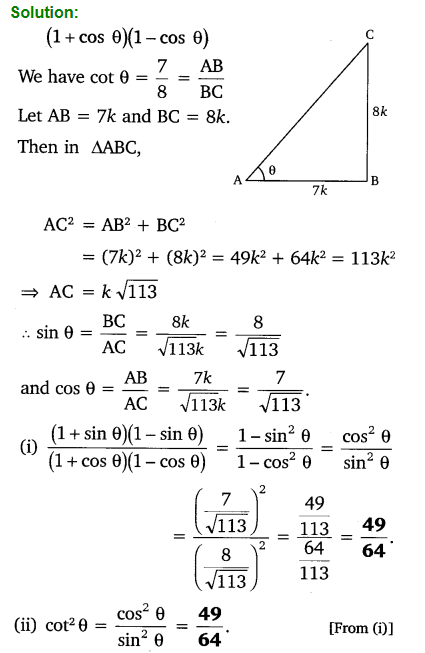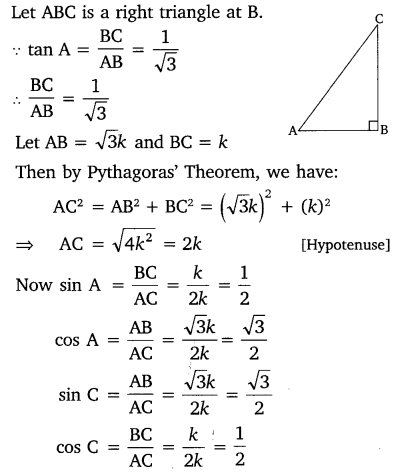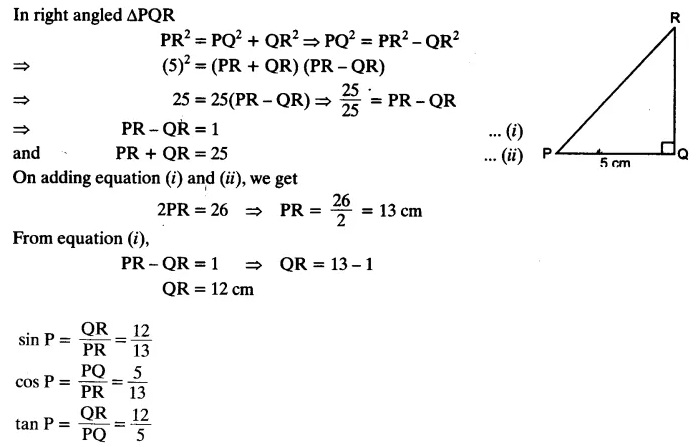India

Introduction:

#### Trigonometry

Trigonometry is the study of relationships between the sides and angles of a right angled triangle.

#### Trigonometric Ratios

Trigonometric ratios of an acute angle in a right triangle express the relationship between the angle and the length of its sides.
Let ∆ABC be a triangle right angled at B. Then the trigonometric ratios of the angle A in right ∆ABC are defined as follows:Note:
The values of the trigonometric ratios of an angle do not vary with the lengths of the sides of the triangle, if the angle remains same.#### Trigonometric Ratios for Complementary Angles

sin (90° – A) = cos A
cos (90° – A) = sin A
tan (90° – A) = cot A
cot (90° – A) = tan A
sec (90° – A) = cosec A
cosec (90° – A) = sec A
Note:
Here (90° – A) is the complementary angle of A.

#### Trigonometric Identities

An equation involving trigonometric ratios of an angle is called a trigonometric identity, if it is true for all values of the angle(s) involved.
(i) sin2θ + cos2θ = 1 [for 0° ≤ θ ≤ 90°]
(ii) sec2θ – tan2θ = 1 [for 0° ≤ θ ≤ 90°]
(iii) cosec2θ – cot2θ = 1 [for 0° < θ ≤ 90°]

Question 1.
In ∆ABC right angled at B, AB = 24 cm, BC = 7 cm. Determine:
(i) sin A, cos A
(ii) sin C, cos C
Solution:Question 2.
In given figure, find tan P – cot R.
Solution:Question 3.
If sin A =$\frac { 3 }{ 4 }$ , calculate cos A and tan A.
Solution:Question 4.
Given 15 cot A = 8, find sin A and sec A.
Solution:Question 5.
Given sec θ =$\frac { 13 }{ 12 }$ , calculate all other trigonometric ratios.
Solution:Question 6.
If ∠A and ∠B are acute angles such that cos A = cos B, then show that ∠A = ∠B.
Solution:Question 7.
If cot θ =$\frac { 7 }{ 8 }$, evaluate:
(i)$\frac { \left( 1+sin\theta \right) \left( 1-sin\theta \right) }{ \left( 1+cos\theta \right) \left( 1-cos\theta \right)}$
(ii) cot²θQuestion 8.
If 3 cot A = 4, check whether$\frac { 1-tan^{ 2 }A }{ 1+tan^{ 2 }A }$ = cos² A – sin² A or not.Question 9.
In triangle ABC, right angled at B, if tan A =$\frac { 1 }{ \surd 3 }$, find the value of:
(i) sin A cos C + cos A sin C
(ii) cos A cos C – sin A sin C
Solution:Question 10.
In ΔPQR, right-angled at Q, PR + QR = 25 cm and PQ = 5 cm. Determine the values of sin P, cos P and tan P.
Solution:Question 11.
State whether the following statements are true or false. Justify your answer.
(i) The value of tan A is always less than 1.
(ii) sec A =$\frac { 12 }{ 5 }$ for some value of angle A.
(iii) cos A is the abbreviation used for the cosecant of angle A.
(iv) cot A is the product of cot and A.
(v) sin θ =$\frac { 4 }{ 3 }$ for some angle.
Solution: